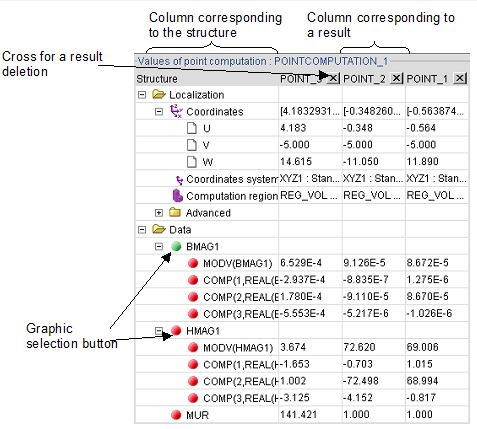# Quantity computation on points: table of results

## Table of results

The numerical results of the computation session are displayed in a table.

## Table of results: overviewElement Function
Column corresponding to the structure The structure of computation point is displayed in the tabulated tree, which one can unfold according to the desired level of information.
Column corresponding to a result point computation is evaluated for each point and a table of result is displayed.
Graphic selection button The user activates the quantity, which is represented graphically while clicking on the red button. The green button marks the graphically displayed quantity.
Cross to delete a result A result can be removed while clicking on the cross.

## Results

Information according to the type of evaluated quantity is provided in the data tree as presented in the table below.

Quantity Restored information
Real scalar The scalar quantity
Complex scalar

The complex modulus

• Real component
• Imaginary component
Real vector

The vector

• The vector modulus
• The 1st component
• The 2nd component
• The 3rd component
Complex vector

The vector at instant t

• The vector complex modulus
• The modulus (1st component)
• The argument (1st component)
• The modulus (2nd component)
• The argument (2nd component)
• The modulus (3rd component)
• The argument (3rd component)